JMSLTM Numerical Library 7.2.0
com.imsl.stat

## Class EmpiricalQuantiles

• All Implemented Interfaces:
Serializable, Cloneable

```public class EmpiricalQuantiles
extends Object
implements Serializable, Cloneable```
Computes empirical quantiles.

The class EmpiricalQuantiles determines the empirical quantiles, as indicated in the array `qProp`, from the data in `x`. The algorithm first checks to see if `x` is sorted; if `x` is not sorted, the algorithm does either a complete or partial sort, depending on how many order statistics are required to compute the quantiles requested. The algorithm returns the empirical quantiles and, for each quantile, the two order statistics from the sample that are at least as large and at least as small as the quantile. For a sample of size n, the quantile corresponding to the proportion p is defined as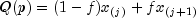where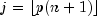,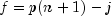, and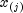, is the j-th order statistic, if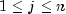; otherwise, the empirical quantile is the smallest or largest order statistic.

Example 1, Serialized Form
• ### Nested Class Summary

Nested Classes
Modifier and Type Class and Description
`static class ` `EmpiricalQuantiles.ScaleFactorZeroException`
The computations cannot continue because a scale factor is zero.
• ### Constructor Summary

Constructors
Constructor and Description
```EmpiricalQuantiles(double[] x, double[] qProp)```
Constructor for `EmpiricalQuantiles`.
• ### Method Summary

Methods
Modifier and Type Method and Description
`double[]` `getQ()`
Returns the empirical quantiles.
`int` `getTotalMissing()`
Returns the total number of missing values.
`double[]` `getXHi()`
Returns the smallest element of `x` greater than or equal to the desired quantile.
`double[]` `getXLo()`
Returns the largest element of `x` less than or equal to the desired quantile.
• ### Methods inherited from class java.lang.Object

`clone, equals, finalize, getClass, hashCode, notify, notifyAll, toString, wait, wait, wait`
• ### Constructor Detail

• #### EmpiricalQuantiles

```public EmpiricalQuantiles(double[] x,
double[] qProp)```
Constructor for `EmpiricalQuantiles`.
Parameters:
`x` - A `double` array containing the data.
`qProp` - A `double` array containing the quantile proportions.
• ### Method Detail

• #### getQ

`public final double[] getQ()`
Returns the empirical quantiles.
Returns:
A `double` array containing the empirical quantiles. `Q[i]` corresponds to the empirical quantile at proportion `qProp[i]`. The quantiles are determined by linear interpolation between adjacent ordered sample values.
• #### getTotalMissing

`public int getTotalMissing()`
Returns the total number of missing values.
Returns:
an `int` scalar value representing the total number of missing values (NaN) in input `x`.
• #### getXHi

`public final double[] getXHi()`
Returns the smallest element of `x` greater than or equal to the desired quantile.
Returns:
A `double` array containing the smallest element of `x` greater than or equal to the desired quantile.
• #### getXLo

`public final double[] getXLo()`
Returns the largest element of `x` less than or equal to the desired quantile.
Returns:
A `double` array containing the largest element of `x` less than or equal to the desired quantile.
JMSLTM Numerical Library 7.2.0